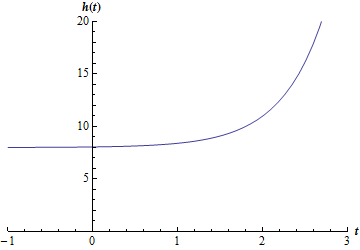Paul's Online Notes
Home / Calculus I / Review / Exponential Functions
Show Mobile Notice Show All Notes Hide All Notes
Mobile Notice
You appear to be on a device with a "narrow" screen width (i.e. you are probably on a mobile phone). Due to the nature of the mathematics on this site it is best views in landscape mode. If your device is not in landscape mode many of the equations will run off the side of your device (should be able to scroll to see them) and some of the menu items will be cut off due to the narrow screen width.

### Section 1.7 : Exponential Functions

3. Sketch the graph of $$h\left( t \right) = 8 + 3{{\bf{e}}^{2t - 4}}$$.

Show Solution

There are several methods that can be used for getting the graph of this function. One way would be to use some of the various algebraic transformations. The point of the problems in this section however are more to force you to do some evaluation of these kinds of functions to make sure you can do them. So, while you could use transformations, we’ll be doing these the “old fashioned” way of plotting points. If you’d like some practice of the transformations you can check out the practice problems for the Common Graphs section of this chapter.

So, with that out of the way here is a table of values for this function.

 $$t$$ -1 0 1 2 3 $$h\left( t \right)$$ 8.0074 8.0549 8.406 11 30.1672

A natural question at this point is “how did we know to use these values of $$t$$”? That is a good question and not always an easy one to answer. For exponential functions the key is to recall that when the exponent is positive the function will grow very quickly and when the exponent is negative the function will quickly get close to zero. This means that often (but not always) we’ll want to keep the exponent in the range of about $$\left[ { - 4,4} \right]$$ and by exponent we mean the value of $$2t - 4$$ after we plug in the $$t$$.

Note that we often won’t need the whole range given above to see what the curve looks like. As we plug in values of $$t$$ we can look at our answers and if they aren’t changing much then we’ll know that the exponent has gone far enough in the negative direction so that the exponential is essentially zero. Likewise, once the value really starts changing fast we’ll know that the exponent has gone far enough in the positive direction as well. The given above is just a way to give us some starting values of $$t$$ and nothing more.

Here is the sketch of the graph of this function.